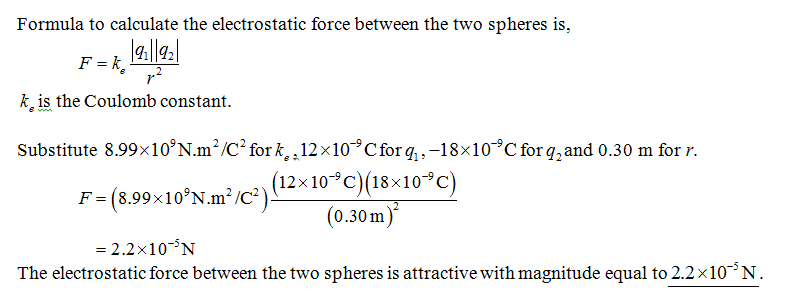# Two small identical conducting spheres are placed with their centers 0.30 m apart. One is given a charge of 12 × 10−9 C, the other a charge of 218 × 10−9 C. (a) Find the electrostatic force exerted on one sphere by the other. (b) The spheres are connected by a conducting wire. Find the electrostatic force between the two after equilibrium is reached, where both spheres have the same charge.

Question
6 views

Two small identical conducting spheres are placed with their centers 0.30 m apart. One is given a charge of 12 × 10−9 C, the other a charge of 218 × 10−9 C. (a) Find the electrostatic force exerted on one sphere by the other. (b) The spheres are connected by a conducting wire. Find the electrostatic force between the two after equilibrium is reached, where both spheres have the same charge.

check_circle

Step 1...

### Want to see the full answer?

See Solution

#### Want to see this answer and more?

Solutions are written by subject experts who are available 24/7. Questions are typically answered within 1 hour.*

See Solution
*Response times may vary by subject and question.
Tagged in